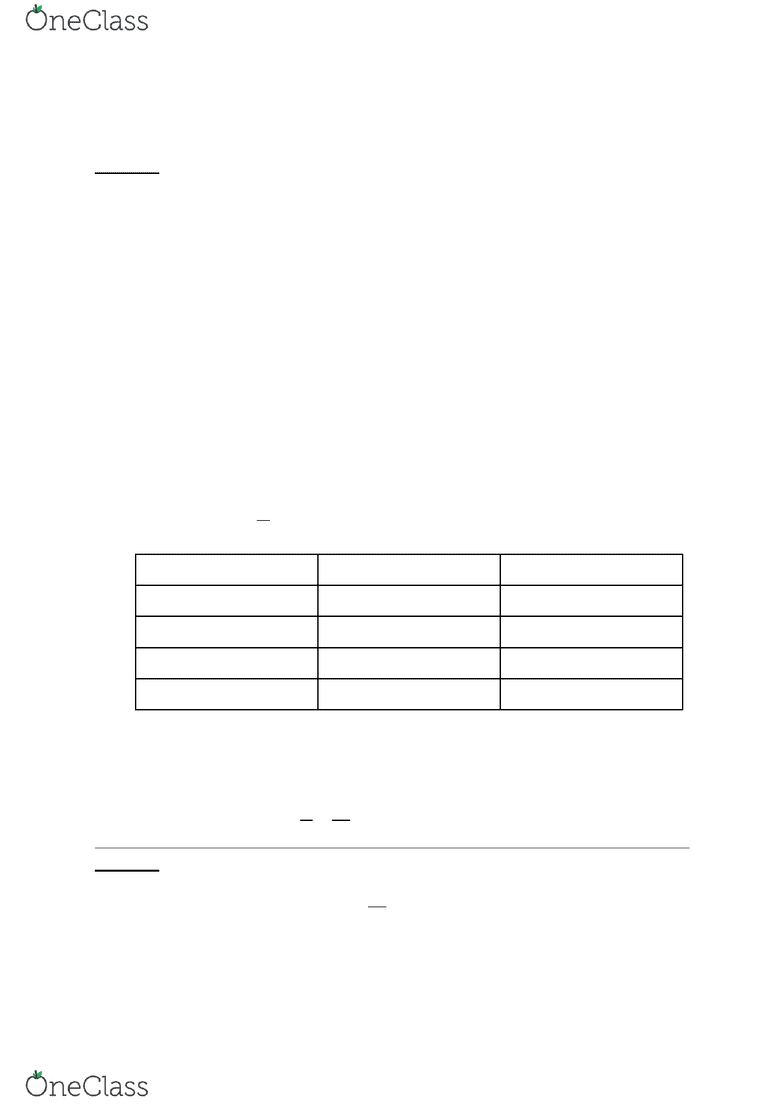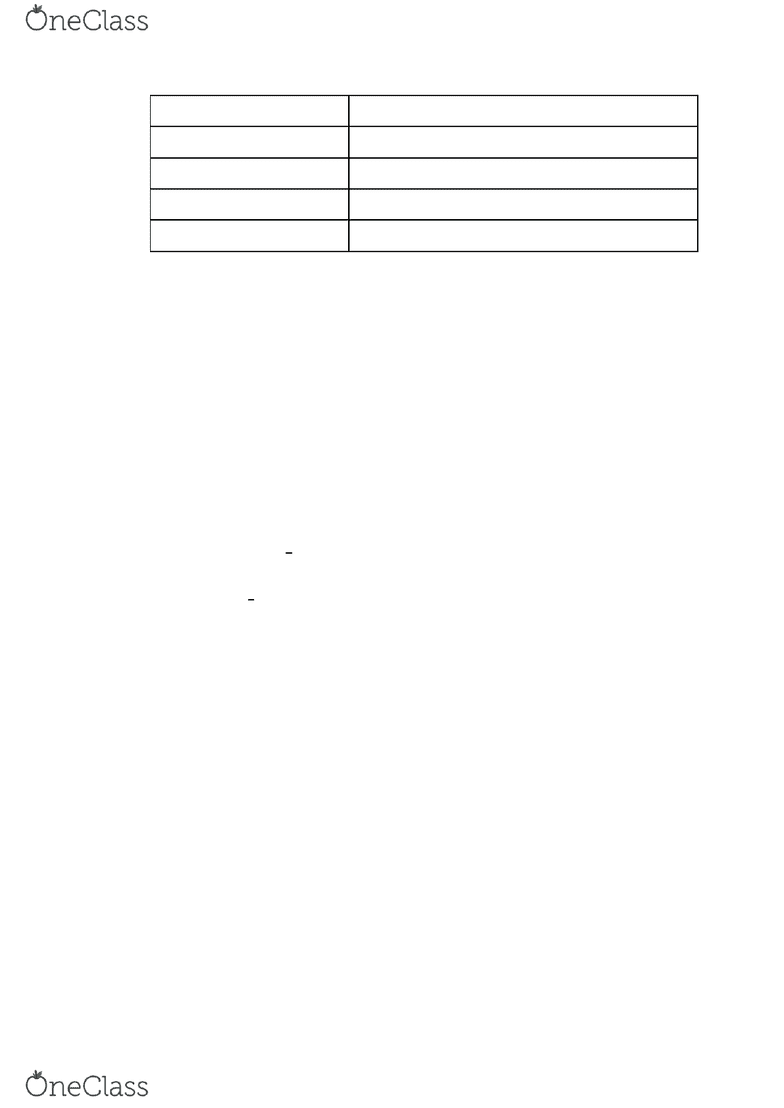# [PHYS 101] - Midterm Exam Guide - Comprehensive Notes for the exam (10 pages long!)

111 views10 pages
School
Department
Course

For unlimited access to Study Guides, a Grade+ subscription is required.Drexel
PHYS 101
MIDTERM EXAM
STUDY GUIDE
Unlock document

This preview shows pages 1-3 of the document.
Unlock all 10 pages and 3 million more documents.

Already have an account? Log inUnlock document

This preview shows pages 1-3 of the document.
Unlock all 10 pages and 3 million more documents.

Already have an account? Log inPHYS 101 LECTURE NOTES WEEK 2
Spencer Kociba
Drexel University
Lecture 3 (01/17/17)
Chapter 2: Motion Along a Straight Line
Dot/Scalar Products
                  
   ,   ,   
Because     so   
         
Vectorial/Cross Products
A x B=C
B x A=-C
      
        
     
Displacement: the vector between the starting and ending point of an object’s path
In a straight line:    and    
Distance: length of the total path traveled
Kinematics: study of motion
Average velocity: 

If the x coordinate is...
Velocity is...
Direction
Positive and increasing
+
+x
Positive and decreasing
-
-x
Negative and increasing
+
+x
Negative and decreasing
-
-x
Instantaneous velocity: velocity at a specific point (of time or position)

Slope of tangent line on an x(t) graph
Acceleration
Average acceleration: rate of change of velocity with time
 



  
Lecture 4 (01/19/17)
Instantaneous acceleration is 
    
    
Signs are the same
    
Signs are the opposite
find more resources at oneclass.com
find more resources at oneclass.com
Unlock document

This preview shows pages 1-3 of the document.
Unlock all 10 pages and 3 million more documents.

Already have an account? Log inIf the x-velocity is
The acceleration is
+ And increasing
+ (moving in +x direction and speeding up)
- And decreasing
- (moving in +x direction and slowing down)
- And increasing
+ (moving in -x direction and slowing down)
- And decreasing
- (moving in -x direction and speeding up)
Slope of velocity is the average x-acceleration
If acceleration is positive, velocity is concave up (increasing)
If acceleration is negative, velocity is concave down (decreasing)
If acceleration is 0, velocity is at a max or a min
It is still possible to be moving with 0 acceleration (you are just moving
at a constant rate)
Motion with constant acceleration
a(t) graph is a horizontal line (slope is 0)
Area under a(t) graph is the change in velocity from time 0 to time t
v(t) graph is a line with a single slope (the x-acceleration)
Area under v(t) graph is the distance traveled (  , the change in
x-coordinate from time 0 to time t
x(t) graph is a parabola
Equations to know
     Includes t, v, and a (no x)
  
Includes t, x, and a (no v)
     Includes x, v, and a (no t)
 
   Includes t, x, and v (no a)
Free fall
Any kind of motion in vertical direction in which the only forces acting on an
object are gravity and air resistance
Air resistance is usually neglected
find more resources at oneclass.com
find more resources at oneclass.com
Unlock document

This preview shows pages 1-3 of the document.
Unlock all 10 pages and 3 million more documents.

Already have an account? Log in

# Get access

Grade+
\$10 USD/m
Billed \$120 USD annually
Homework Help
Class Notes
Textbook Notes
40 Verified Answers
Study Guides
1 Booster Class